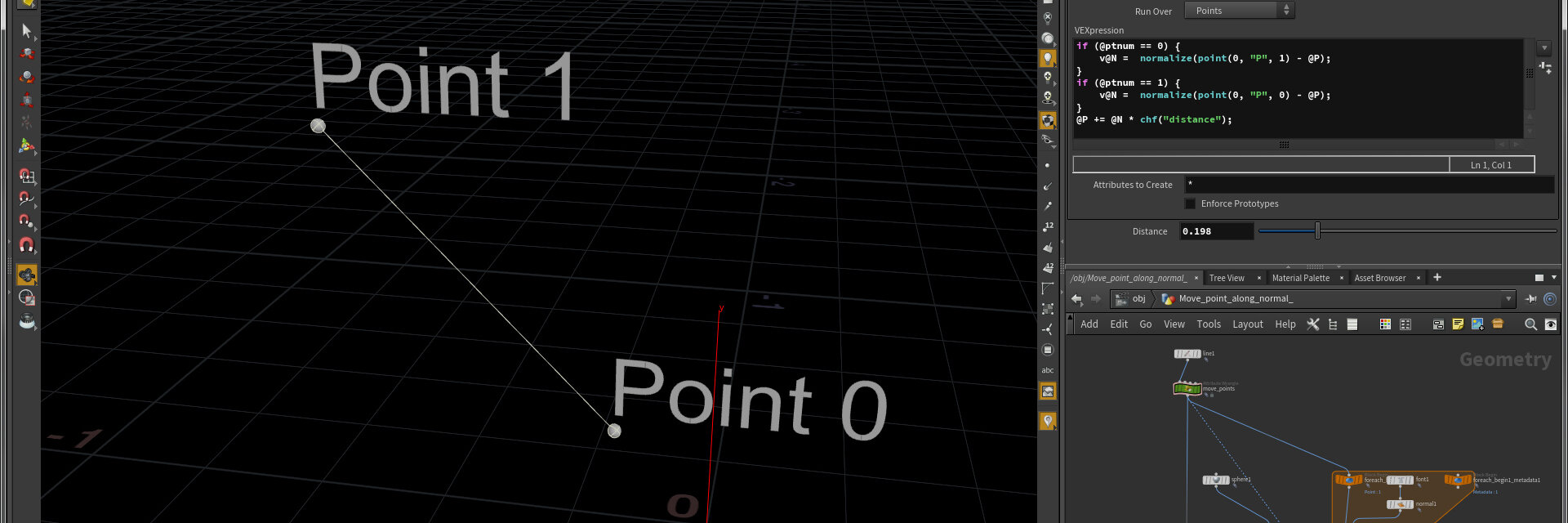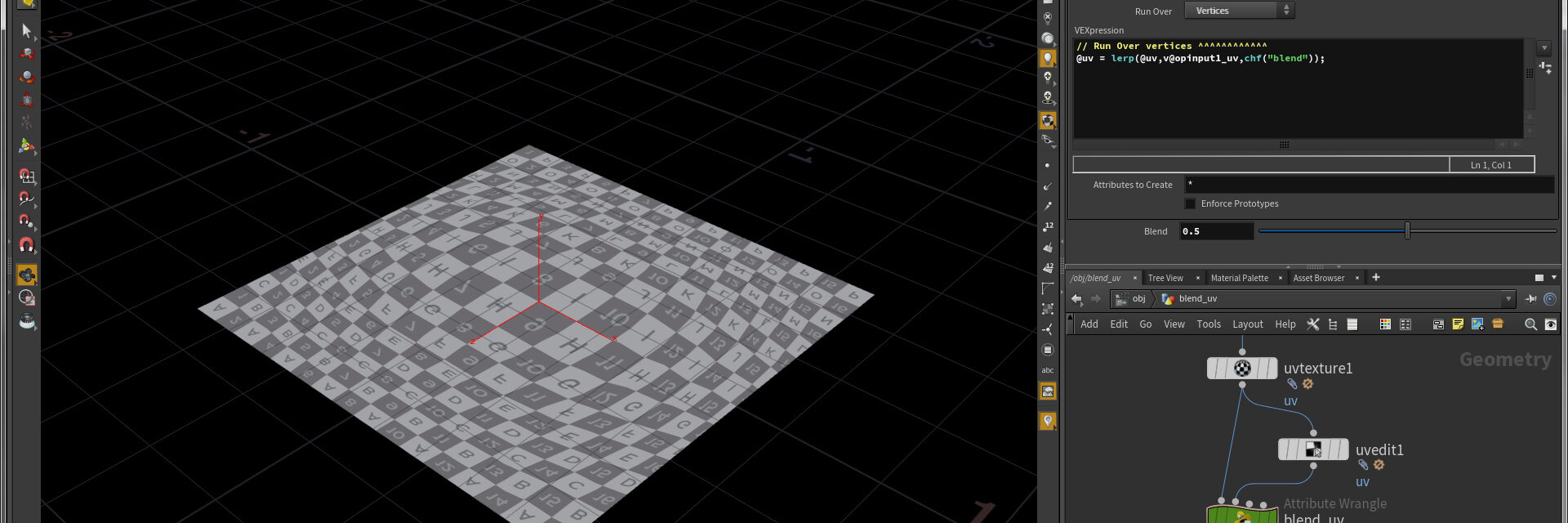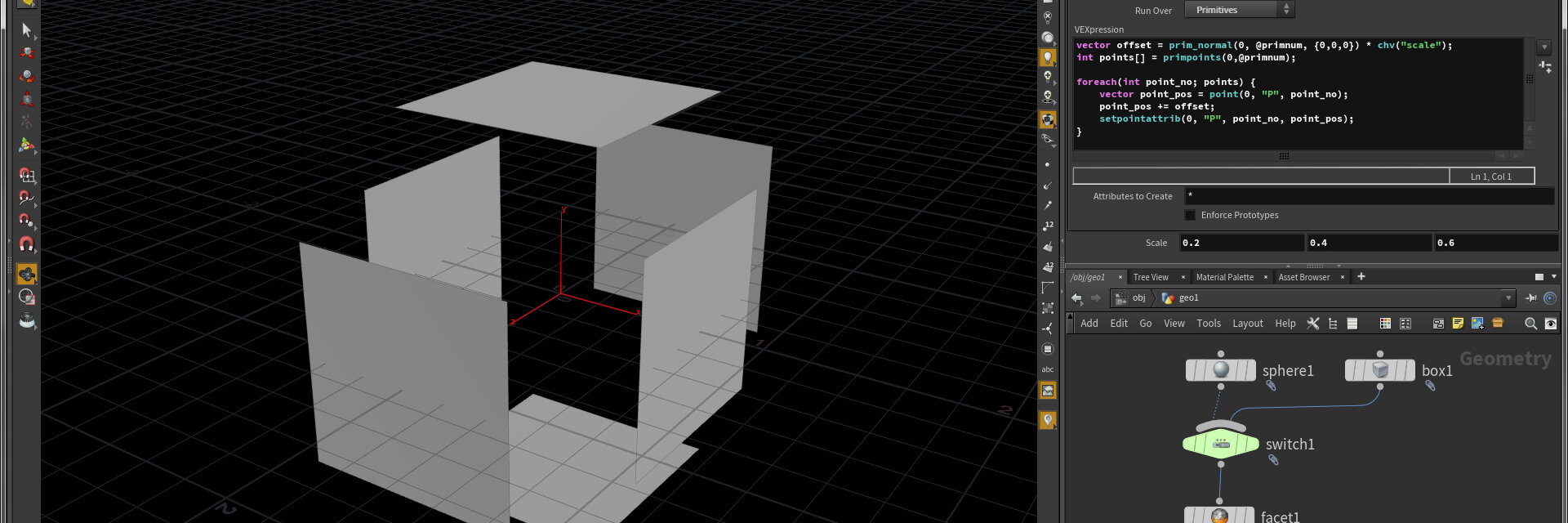(Houdini, Vex)

Small reminder code snippets

# Houdini Snippets

A list with some small Houdini Wrangle Snippets.

#### VEX Move pointHoudini, Vex |

Move point along a given vector

## Move point along normal

Create a line, then add a point wrangle with the following code and create the spare parameters.

Move Point along Normal

``````if (@ptnum == 0) {
v@N = normalize(point(0, "P", 1) - @P);
}
if (@ptnum == 1) {
v@N = normalize(point(0, "P", 0) - @P);
}
@P += @N * chf("distance");``````

#### VEX Blend UVsHoudini, Vex |

Blend/lerp attributes

## How to Blend Attributes / UVs in Houdini

Use this code in a Point Wrangle. Create UVs and a UV Edit Node. Assign original UVs to first input and the edited UVs as second input. Set Run Over to Vertices, if you have vertex uvs. ... read more

Blend UVs

``````// Set run over to type with the attribute
@uv = lerp(@uv,v@opinput1_uv,chf("blend"));``````

#### VEX Move PrimitiveHoudini, Vex |

Move primitive along a given vector

## Move Primitive along Normal

Create a Box or Sphere and set Primitive Type to Polygon Mesh. Create a facet Node with "Unique Points" checked to create separated Prims. Create a Primitive Wrangle with this snippet and create the Spare Parameter.

Move Primitive

``````vector offset = prim_normal(0, @primnum, {0,0,0}) * chv("scale");
int points[] = primpoints(0,@primnum);

foreach(int point_no; points) {
vector point_pos = point(0, "P", point_no);
point_pos += offset;
setpointattrib(0, "P", point_no, point_pos);
}``````

#### VEX Spherize a BoxHoudini, Vex |

## Spherize a Box

Create a box and set Primitive Type to Polygon Mesh. Set Axis Divisions to maybe 10. Create a Point Wrangle with this snippet and create the Spare Parameter.

Spherize a Box

``````vector min, max;
getbbox(0, min, max);
vector center = (min+max)/2;
@P = chf("radius") * normalize(@P - center) + center;``````

#### VEX Rotate around EdgeHoudini, Vex |

Rotate Primitive Points around an Edge

## Rotate around Edge

Create a Box or Sphere and set Primitive Type to Polygon Mesh. Create a facet Node with "Unique Points" checked to create separated Prims. Create a Point Wrangle with this snippet and create the Spare Parameter.

Rotate around Edge

``````float angle = radians(chf("angle"));
matrix3 rotm = ident();
int points[] = primpoints(0, @primnum);
vector p0 = point( 0, "P", points );
vector p1 = point( 0, "P", points );
vector axis = normalize( p0 - p1 );

rotate(rotm, angle, axis);

@P -= p0;
@P *= rotm;
@P += p0;``````

#### VEX Create PrimitiveHoudini, Vex |

Simpe Snippet Code to create a primitive

## Create Primitive Circle

Create a Attribute Wrangle (Run Over: Detail) with this snippet and create the Spare Parameter. Maybe change the "close_poly" and "add_end" parameters to Type "Toggle" manually.

Create Circle

``````// define pi or use \$PI
float pi = 3.14159265358979323846;
// prepare parameters
int close = chi("close_poly");
float count = chi("point_count") + add_end;
float mul = (count - add_end) / pi / 2; // * 4
// create empty arrayfor points
int pts[];
// create points and add them to point array
for (int i = 0; i < count; i++) {
float x = sin(i/mul);
float z = cos(i/mul);
push(pts,pt);
}
// create open or closed primitve
if (close) {
} else {
}``````

#### VEX Create Vertex UVsHoudini, Vex |

Create a simple Primitive with Vertex UVs

## Create Vertex UVs

Create an Attribute Wrangle (Run Over: Detail) with this snippet and create the Spare Parameters.

Create Vertex UVs

``````// create parameters
float _x = chf("width");
float _z = chf("height");
// create point positions
vector _pos[] = array({0,0,0},set(_x,0,0),set(_x,0,_z),set(0,0,_z));
// create empty point array
int _pts[];
// loop over point positions and create the points
foreach (vector _pt_pos; _pos) {